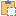#### AreaReinforcementCurve Class

An object that specifies the type of a floor in Autodesk Revit.

Namespace: Autodesk.Revit.DB.Structure
Assembly: RevitAPI (in RevitAPI.dll) Version: 21.0.0.0 (21.1.1.109)

# Syntax

C#
``public class AreaReinforcementCurve : CurveElement``
Visual Basic
``````Public Class AreaReinforcementCurve _
Inherits CurveElement``````
Visual C++
``public ref class AreaReinforcementCurve : public CurveElement``

# Remarks

The structural layers of the floor can be accessed via this object.

# ExamplesCopy C#
``````public void GetInfo_AreaReinforcementCurve(AreaReinforcement areaReinforcement)
{
string message = "Area Reinforcement Curves : ";

// Get area reinforcement curves by iterating the Curves property
IList<ElementId> curveIds = areaReinforcement.GetBoundaryCurveIds();
foreach (ElementId ii in curveIds)
{
AreaReinforcementCurve areaReinforcementCurve = doc.GetElement(ii) as AreaReinforcementCurve;
if (null == areaReinforcementCurve)
{
continue;
}
Autodesk.Revit.DB.Curve curve = areaReinforcementCurve.Curve;

// Get curve start point
message += "\nCurve start point:(" + curve.GetEndPoint(0).X + ", "
+ curve.GetEndPoint(0).Y + ", " + curve.GetEndPoint(0).Z + ")";
// Get curve end point
message += "\nCurve end point:(" + curve.GetEndPoint(1).X + ", "
+ curve.GetEndPoint(1).Y + ", " + curve.GetEndPoint(1).Z + ")";
}

}``````Copy VB.NET
``````Public Sub GetInfo_AreaReinforcementCurve(areaReinforcement As AreaReinforcement)
Dim message As String = "Area Reinforcement Curves : "

' Get area reinforcement curves by iterating the Curves property
Dim curveIds As IList(Of ElementId) = areaReinforcement.GetBoundaryCurveIds()
For Each ii As ElementId In curveIds
Dim areaReinforcementCurve As AreaReinforcementCurve = TryCast(doc.GetElement(ii), AreaReinforcementCurve)
If areaReinforcementCurve Is Nothing Then
Continue For
End If
Dim curve As Autodesk.Revit.DB.Curve = areaReinforcementCurve.Curve

' Get curve start point
message += ((vbLf & "Curve start point:(" + curve.GetEndPoint(0).X & ", ") + curve.GetEndPoint(0).Y & ", ") + curve.GetEndPoint(0).Z & ")"
' Get curve end point
message += ((vbLf & "Curve end point:(" + curve.GetEndPoint(1).X & ", ") + curve.GetEndPoint(1).Y & ", ") + curve.GetEndPoint(1).Z & ")"
Next